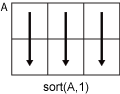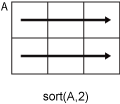# sort

## 语法

``B = sort(A)``
``B = sort(A,dim)``
``B = sort(___,direction)``
``B = sort(___,Name,Value)``
``[B,I] = sort(___)``

## 说明

``B = sort(A)` 按升序对 `A` 的元素进行排序。如果 `A` 是向量，则 `sort(A)` 对向量元素进行排序。如果 `A` 是矩阵，则 `sort(A)` 会将 `A` 的列视为向量并对每列进行排序。如果 `A` 是多维数组，则 `sort(A)` 沿大小不等于 1 的第一个数组维度计算，并将这些元素视为向量。`

``B = sort(A,dim)` 返回 `A` 沿维度 `dim` 的排序元素。例如，如果 `A` 是一个矩阵，则 `sort(A,2)` 对每行中的元素进行排序。`

``B = sort(___,direction)` 使用上述任何语法返回按 `direction` 指定的顺序显示的 `A` 的有序元素。`'ascend'` 表示升序（默认值），`'descend'` 表示降序。`

``B = sort(___,Name,Value)` 指定用于排序的其他参数。例如，`sort(A,'ComparisonMethod','abs')` 按模对 `A` 的元素进行排序。`

``[B,I] = sort(___)` 还会为上述任意语法返回一个索引向量的集合。`I` 的大小与 `A` 的大小相同，它描述了 `A` 的元素沿已排序的维度在 `B` 中的排列情况。例如，如果 `A` 是一个向量，则 `B = A(I)`。`

## 示例

```A = [9 0 -7 5 3 8 -10 4 2]; B = sort(A)```
```B = 1×9 -10 -7 0 2 3 4 5 8 9 ```

`A = [3 6 5; 7 -2 4; 1 0 -9]`
```A = 3×3 3 6 5 7 -2 4 1 0 -9 ```
`B = sort(A,2)`
```B = 3×3 3 5 6 -2 4 7 -9 0 1 ```

`A = [10 -12 4 8; 6 -9 8 0; 2 3 11 -2; 1 1 9 3]`
```A = 4×4 10 -12 4 8 6 -9 8 0 2 3 11 -2 1 1 9 3 ```
`B = sort(A,'descend')`
```B = 4×4 10 3 11 8 6 1 9 3 2 -9 8 0 1 -12 4 -2 ```

```A = ["Santos","Burns"; ... "Jones","Morita"; ... "Petrov","Adams"]; B = sort(A)```
```B = 3x2 string "Jones" "Adams" "Petrov" "Burns" "Santos" "Morita" ```

`B = sort(A,2)`
```B = 3x2 string "Burns" "Santos" "Jones" "Morita" "Adams" "Petrov" ```

```ds = {'2012-12-22';'2063-04-05';'1992-01-12'}; A = datetime(ds,'Format','yyyy-MM-dd')```
```A = 3x1 datetime 2012-12-22 2063-04-05 1992-01-12 ```
`[B,I] = sort(A)`
```B = 3x1 datetime 1992-01-12 2012-12-22 2063-04-05 ```
```I = 3×1 3 1 2 ```

`B` 列出排序后的日期以及包含 `A` 的相应索引的 `I`

`A(I)`
```ans = 3x1 datetime 1992-01-12 2012-12-22 2063-04-05 ```

```X = [3 6 4 2 1 5]; Y = ["yellow" "purple" "green" "orange" "red" "blue"];```

`[Xsorted,I] = sort(X)`
```Xsorted = 1×6 1 2 3 4 5 6 ```
```I = 1×6 5 4 1 3 6 2 ```
`Ysorted = Y(I)`
```Ysorted = 1x6 string "red" "orange" "yellow" "green" "blue" "purple" ```

```A(:,:,1) = [2 3; 1 6]; A(:,:,2) = [-1 9; 0 12]; A```
```A = A(:,:,1) = 2 3 1 6 A(:,:,2) = -1 9 0 12 ```
`B = sort(A,3)`
```B = B(:,:,1) = -1 3 0 6 B(:,:,2) = 2 9 1 12 ```

`B = sort(A(:))`
```B = 8×1 -1 0 1 2 3 6 9 12 ```

```A = [1+2i 3+1i 1i 0 -1i]; B = sort(A,'ComparisonMethod','real')```
```B = 1×5 complex 0.0000 - 1.0000i 0.0000 + 0.0000i 0.0000 + 1.0000i 1.0000 + 2.0000i 3.0000 + 1.0000i ```

## 输入参数

• 如果 `A` 是标量，则 `sort(A)` 返回 `A`

• 如果 `A` 是复数，默认情况下，`sort` 会按模对元素进行排序。如果有多个元素具有相等的模，则按区间 (−π, π] 上的相位角对这些元素进行排序。

• 如果 `A` 是字符向量元胞数组或字符串数组，则 `sort(A)` 按照 UTF-16 字符编码方案的代码顺序对元素进行排序。排序区分大小写。有关对字符和字符串数组进行排序的详细信息，请参阅字符和字符串数组的排序顺序

• 如果 `A` 是字符串数组，则 `sort` 将对数组元素重新排序，但不会对字符串中的字符重新排序。

• 如果 `A` 是分类数组，则排序顺序基于 `categories(A)` 返回的类别顺序。

• 假定有矩阵 `A``sort(A,1)``A` 的列元素进行排序。• `sort(A,2)``A` 的行元素进行排序。### 名称-值参数

• `'auto'` - 缺失的元素放在最后（对于升序排序）或放在最前面（对于降序排序）。

• `'first'` - 缺失的元素放在最前面。

• `'last'` - 缺失的元素放在最后。

• `'auto'` - 当 `A` 为实数时，按 `real(A)``A` 进行排序；当 `A` 为复数时，按 `abs(A)` 进行排序。

• `'real'` - 当 `A` 为实数或复数时，按 `real(A)``A` 进行排序。如果 `A` 包含具有相等实部的元素，则使用 `imag(A)` 进行排序。

• `'abs'` - 当 `A` 为实数或复数时，按 `abs(A)``A` 进行排序。如果 `A` 包含具有相等模的元素，则使用区间 (-π,π] 中的 `angle(A)` 进行排序。

## 输出参数

`sort` 函数使用一种稳定的排序算法。因此，当输入包含重复值时，无论排序方向如何，排序索引都会保留输入的原始顺序。例如，如果 `A = [1 2 1 2]`，则 `[Ba,Ia] = sort(A,'ascend')` 返回排序索引 `Ia = [1 3 2 4]``[Bd,Id] = sort(A,'descend')` 返回排序索引 `Id = [2 4 1 3]`

## 详细信息

### 字符和字符串数组的排序顺序

MATLAB® 使用 UTF-16 字符编码方案将字符存储为 Unicode®。字符和字符串数组按 UTF-16 代码点顺序进行排序。对于同时也是 ASCII 字符的字符，此顺序意味着大写字母在小写字母之前。数字和某些标点符号也在字母之前。

## 提示

• `sortrows` 函数提供了额外的灵活性，可基于矩阵或表输入的多列进行子排序。

• `sort` 函数和关系运算符对复数使用不同顺序。有关详细信息，请参阅关系运算